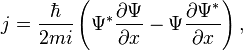# What is the physical dimension/unit of Probability current?

• EhrEnFeest

## Homework Statement

Question:
What is the physical dimension of Probability Current for a particle in 1 dimension? (Quantum Mechanics)

## Homework Equations

Quantum mechanical Probability Current:## The Attempt at a Solution

I know the physical dimension of mass, that is kg. If I know every dimension, I can try some things and I can find the dimension of the observable. But now, I'm stuck. I guess that the wave function has no dimension, because it is very related to probability. But what's the case with $$\frac{\partial \Psi}{\partial x}$$?

Hello Feest,m-1 I would dare to venture ... (on your last question)

Hello Feest,m-1 I would dare to venture ... (on your last question)
But h has dimension of action. Taking Ψ to be dimensionless, j would appear to have dimension of velocity.

Actually ##j## is a probability current density.

Actually ##j## is a probability current density.
Just realized you meant m as metres, not mass. The question is for dimension, so I would answer L-1 there.
But what do you think the dimension of j is, if not LT-1?

With $${\partial \rho\over \partial t} + \nabla\cdot {\bf j} = 0$$ where ##\rho = \psi^*\psi ## and ##\int \rho \, d\tau = 1##, I would end up with L-2T-1And now I have to backtrack to 1 dimension as cleary stated in post #1 () ending up with T-1I guess that the wave function has no dimension, because it is very related to probability.
So here we must react also: It is related to probability in the sense that $$\int \psi^*\psi\,d\tau = 1$$where the integral is over all space. The ##1## is dimensionless: a genuine probability. So guess again !

•EhrEnFeest
So here we must react also: It is related to probability in the sense that $$\int \psi^*\psi\,d\tau = 1$$where the integral is over all space. The ##1## is dimensionless: a genuine probability. So guess again !

This is a nice question to answer. It took me some time but I think:
∫ψ∗ψdτ=1 has no dimensions.
But as you integrate over space, and in this case this is one-dimensional, the dimension of ψ∗ψ is multiplied with [length]. And then ψ has a dimension of [(1/length)^½]?

An unavoidable conclusion, isn't it ? I never worried about the wave function having a dimension (always considered it as dimensionless) and would have liked to keep it that way. But -- unless we are being corrected -- this is what comes out !

So thank you everyone!

Conclusion: the dimension of the probability current is [1/time]?

These physical dimensions used to help me give interpretations to different physical quantities. But in QM, that's not (yet) the case. :)